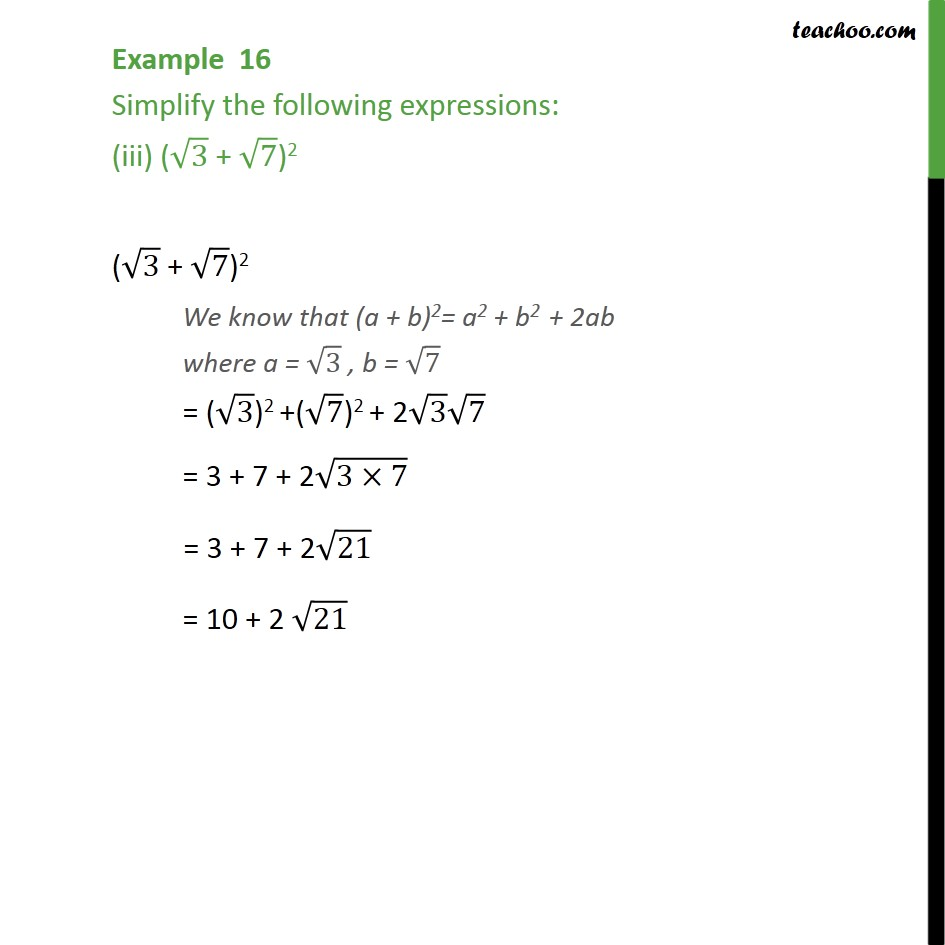Examples

Chapter 1 Class 9 Number Systems
Serial order wiseGet live Maths 1-on-1 Classs - Class 6 to 12

### Transcript

Example 16 Simplify the following expressions: (iii) ( 3 + 7)2 ( 3 + 7)2 We know that (a + b)2= a2 + b2 + 2ab where a = 3 , b = 7 = ( 3)2 +( 7)2 + 2 3 7 = 3 + 7 + 2 (3 7) = 3 + 7 + 2 21 = 10 + 2 21